# Hnco-ge-relax fully relaxed accordion HNCO (Tjandra 2009)

(diff) ←Older revision | Current revision (diff) | Newer revision→ (diff)

This page is a part of the user-editable NMR Wiki pulse sequence database.

Disclaimer: You are solely responsible for correct use of these materials on your instrument.

Synopsis: uses line-width in a single 3D spectrum to simultaneously measure 15N T1 and T2 relaxation rates in proteins

Family: HNCO

 Code authors: Nico Tjandra, Kang Chen

Copyright: Nico Tjandra, National Institutes of Health

The pulse sequence is based on a 3D HNCO experiment, where indirect amide 15N and carbonyl 13C dimensions are recorded in constant time mode. The key modification is adding T1 and T2 relaxation loops to the sequence prior to the indirect evolution delays.

In the constant time evolution experiments, indirect dimension FIDs have periodic profile with no decay. However this pulse sequence introduces decay to those profiles, corresponding to T1 and T2 relaxation processes, therefore relaxation rates can be extracted directly from the line width after the fourier transformation.

Length of the relaxation delay is incremented simultaneously with the corresponding (indirect dimension) evolution delay, i.e. in the accordion fashion. Therefore, the amount of relaxation in a given indirect FID is proportional to the indirect evolution time, yielding the exponentially decaying profile.

The chemical shift and relaxation evolve at different time scales, because the corresponding time increments are not equal. So in order to extract the relaxation time, a scaling factor between the two increments needs to be taken into account.

Relaxation time Tr can be calculated as: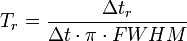$T_r=\frac{\Delta t_r}{\Delta t \cdot \pi \cdot FWHM}$

Here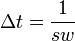$\Delta t = \frac{1}{sw}$, and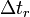$\displaystyle \Delta t_r$ is the relaxation delay increment.

Section on dimensions explains how to calculate the scaling factor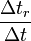$\frac{\Delta t_r}{\Delta t}$.

The two indirect dimensions in this experiment simultaneously record chemical shift and relaxation rates. The last section below explains how to calculate the scaling factors.

Spectral window and relaxation delay increment are hardcoded in both dimensions.

#### δN (amide),T2 or T1 dimension

• in22=in24=in25=1/(4*swN), where swN - spectral window in 15N dimension
• T2 loop increment$\displaystyle \Delta t_r$: 24*d13 (12 ms)
• loop counter NLOOP - hardcoded number of complex points
• #define T2 turns on T2 loop
• #define T1_onN switches this dimension to record T1 relaxation
• detection mode: Echo-Antiecho

#### Calculation of scaling factors

Scaling factors$\frac{\Delta t_r}{\Delta t}$ are calculated as follows:

 factor formula default value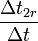$\frac{\Delta t_{2r}}{\Delta t}$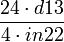$\frac{24 \cdot d13}{4 \cdot in22}$ 21.43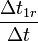$\frac{\Delta t_{1r}}{\Delta t}$ if #define T1_onN is uncommented and #define T2 is commented out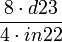$\frac{8 \cdot d23}{4 \cdot in22}$ 57.14$\frac{\Delta t_{1r}}{\Delta t}$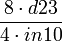$\frac{8 \cdot d23}{4 \cdot in10}$ 50
• definition: channel H
• carrier: ~ 4.8 ppm (H2O)
• p1 @ pl1 - high power 90o 1H pulse
• decoupling cpds1: p30 @ pl30 - 90o pulse (60 μs for 600 MHz)

Two 13C channels are used in the sequence: one for Cα and one for C' (carbonyl). Channel definitions are set in file bits.nt. You might have to tweak that file and the frequency offsets for the shaped pulses if you have only one channel for 13C.

#### Cα

• definition: channel C1
• carrier: ~56 ppm (center of Cα region)
• p4 @ pl4 - soft 180o Cα pulse (with null @ CO) 47.4 μs for 600 MHz

#### C'

• definition: channel C2
• carrier: ~177 ppm (center of C' - carbonyl - region)
• shaped pulse sp1: p6, sinc. 180o pulse on C', 191.7 μs for 600 MHz
• shaped pulse sp2: p6, sinc. 90o pulse on C', 191.7 μs for 600 MHz
• definition: channel N
• carrier: ~115 ppm (center of amide nitrogen region)
• p7 @ pl7 - high power 90o 15N pulse
• decoupling cpd2: p31 @ pl31 - soft 90o 15N pulse for waltz16, 190 μs for 600 MHz

Durations of gradient pulses are hard-coded in the program

All gradients are sine-shaped, except gp24-27, which are square.

Tune p26 or p24/p25, p24+p25=p26.

• p19:gp19, 20% on X
• p21:gp21, 25% on Y
• p22:gp22, 30% on X
• p23:gp23, 30% on Y
• p24:gp24, square 30% on Z
• p25:gp25, square -30% on Z
• p26:gp26, square -30% on Z
• p26:gp27, square 30% on Z
• p29:gp29, 30% on Y
• #define HEAT - turns on sample heating compensation loop
• #define NITRO - ???